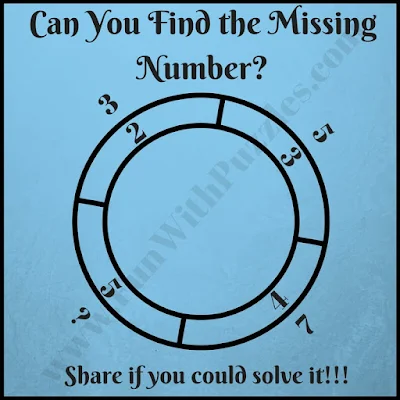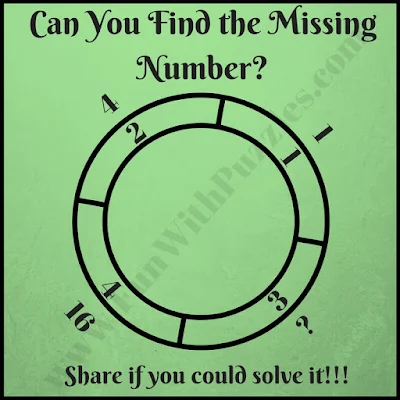Math Brain Teasers can be created using different shapes. Earlier we have published puzzles with Square Math Brain Teasers in which there are four squares contains the number with relation to each other with some mathematical relationship, Circle Math Brain Teasers and Star Math Brain Teasers in which numbers are around Circle and Star respectively, and one has to find the missing numbers while finding the relationship among other numbers. Today we are posting Numbers in Double Circle Math Brain Teasers.
In these Math Brain Teasers, there are two concentric circles that are divided into four segments. Each of these segments contains one number inside and outside the outer circle. All the number given in these double circle contains some mathematical pattern or relationship with each other. One has to find this mathematical pattern or sequence and then find the missing number which will replace the question mark.
So are you ready to solve these Double Circle math brain teasers? These are easy maths logic puzzles with everyone from kids to teens and adults should be able to solve these puzzles very quickly. Do write your solutions in the comment section of this post.1. What will replace the question mark riddle2. Easy math double circle picture puzzle3. Fun math double circle picture puzzle4. Quick math double circle riddle5. Tricky math double circle brain teaser